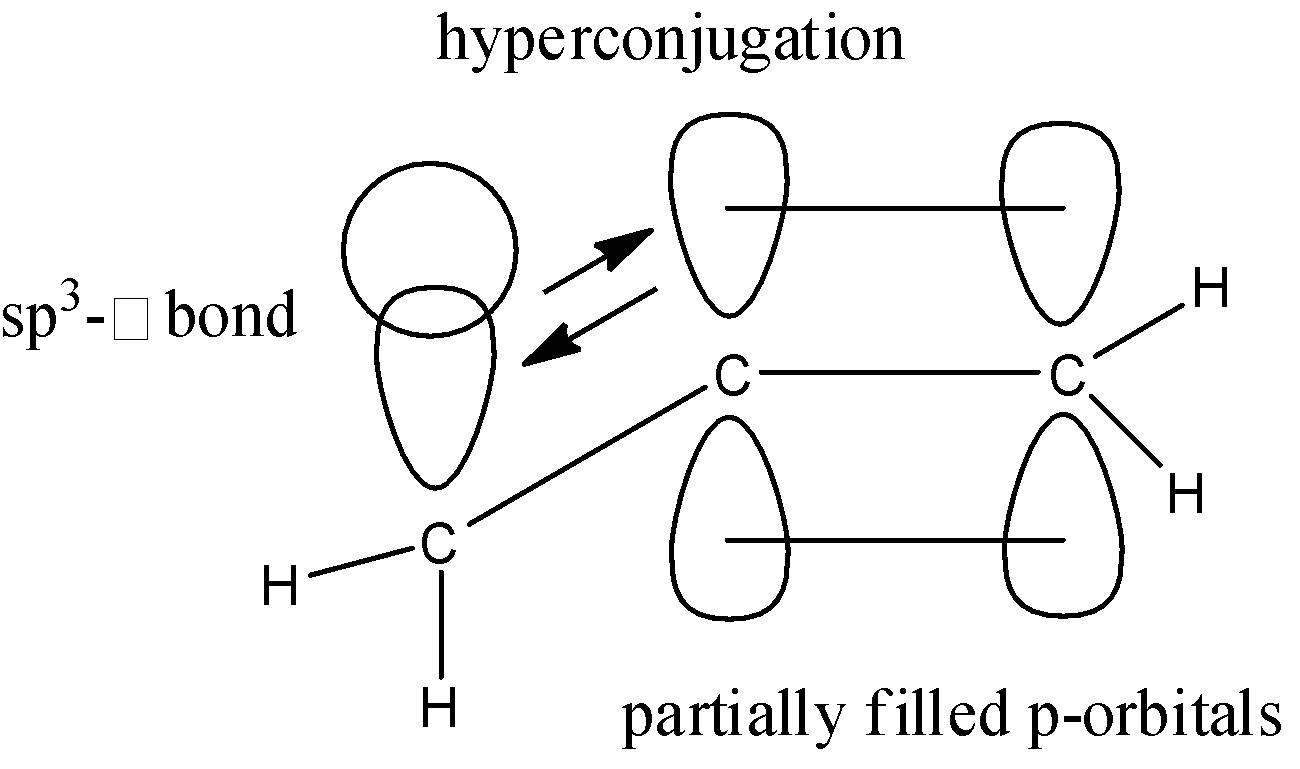QuestionAnswers

# An alkyl group acts as _______ when attached to a $\pi$- system.A. electron acceptorsB. electron donorsC. hydrogen donorsD. do not interact with the $\pi$- system

Hint: Think about the type of bonds that alkyl groups form and how these bonds may act in hyperconjugation with the adjacent empty or partially filled p-orbital which is present in a $\pi$- system.

Complete solution:
We know that, in a $\pi$- system the bond formation takes place due to the sideways overlap of the p-orbitals. We also have an $s{{p}^{3}}-\sigma$ bond in the same plane as the $\pi$- bond. This $s{{p}^{3}}-\sigma$ bond delocalizes the electrons in the $\pi$- system and causes hyperconjugation. This hyperconjugation bring about stability by extending the molecular orbital. Let us take an example to understand what hyperconjugation really means. Consider the propene molecule:Here, we can see that the $s{{p}^{3}}-\sigma$ bond from the methyl group, delocalized the electrons in the partially filled p-orbitals. This process is known as hyperconjugation and works on the principle of donation of electrons.
Here note that the hydrogen atom that should be present on the central carbon atom is not given due to constraint of space; assume that it is present at the back of the molecule.
The resonance structures of the molecule are:Here, we can see that the resonance due to hyperconjugation gives the structure some stability. This stability is seen due to the electron donating effect of the methyl group.
We can generalize the example given above and say that all alkyl groups are capable of providing electrons to the $\pi$- system and acting as electron donors. This kind of hyperconjugation is possible even if there is a $s{{p}^{3}}-s{{p}^{3}}$ bond present.

Hence, the correct answer is ‘B. electron donors’

Note:
The alkyl group cannot be an electron acceptor as all the atoms involved are too electropositive for that to happen. An electron accepting group may also cause similar resonance structures. The donation of hydrogen atoms doesn’t occur in the same molecule here and cannot be an interaction between the alkyl group and the $\pi$- system. The interaction between them is essential as they are usually present in the same plane.